Ex 13.3

Chapter 13 Class 9 Surface Areas and Volumes
Serial order wise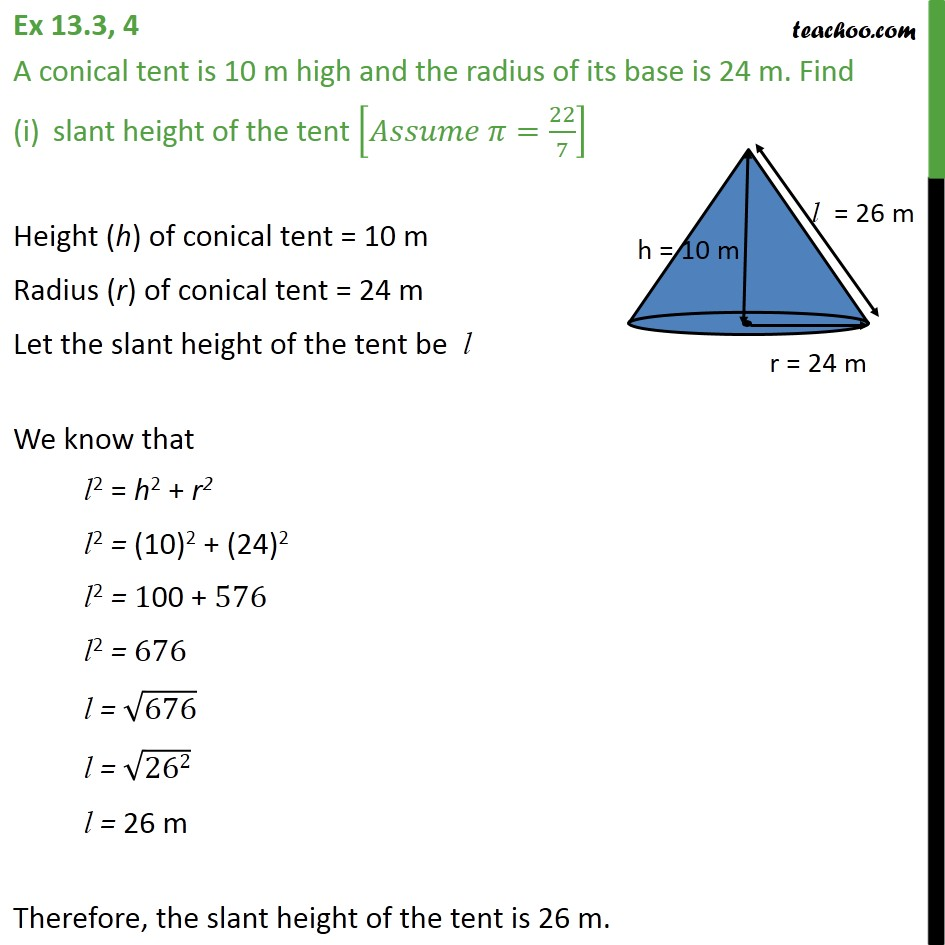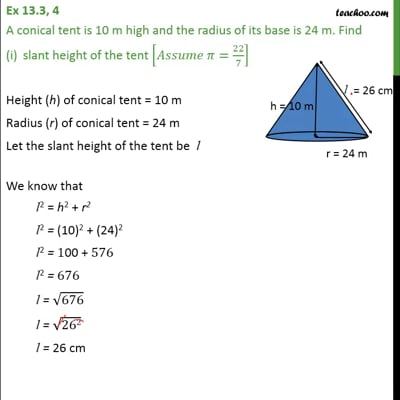This video is only available for Teachoo black users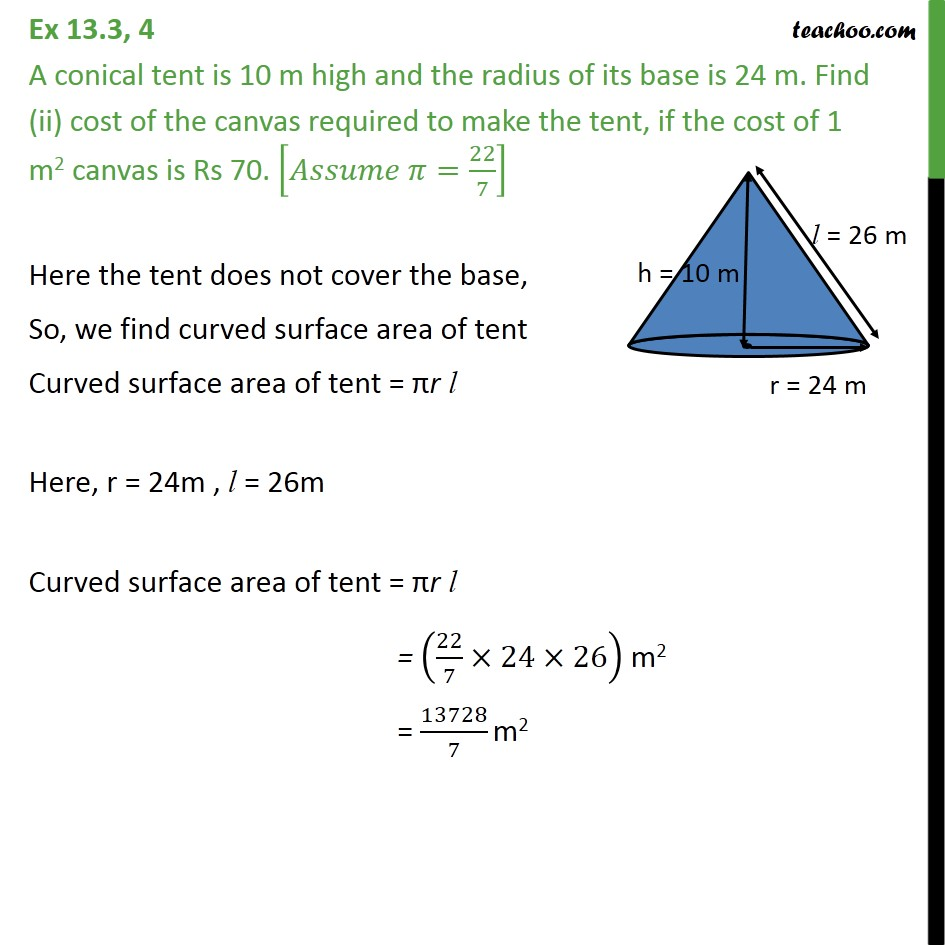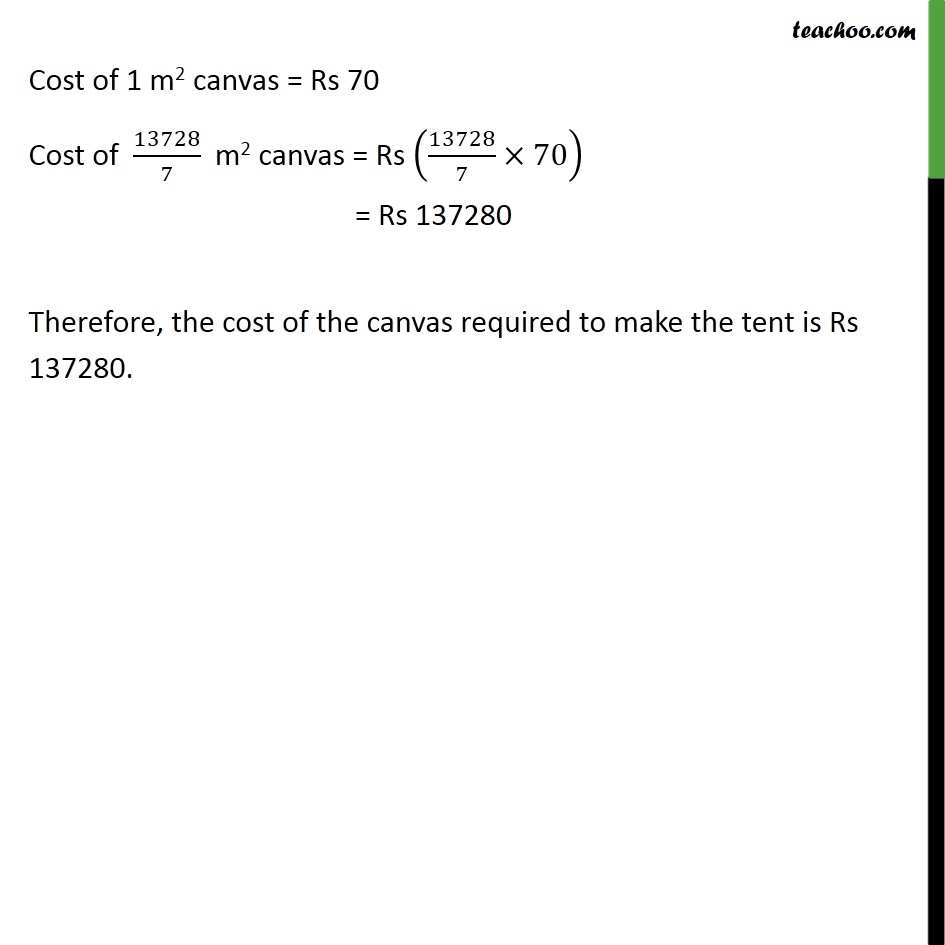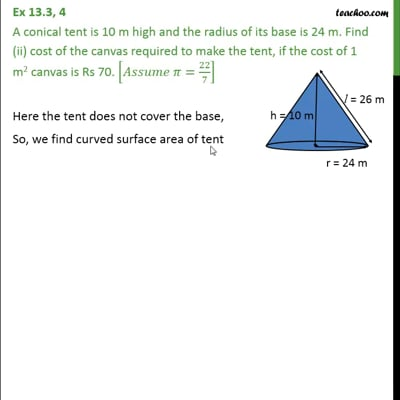This video is only available for Teachoo black users

Solve all your doubts with Teachoo Black (new monthly pack available now!)

### Transcript

Ex 13.3, 4 A conical tent is 10 m high and the radius of its base is 24 m. Find slant height of the tent [𝐴𝑠𝑠𝑢𝑚𝑒 𝜋=22/7] Height (h) of conical tent = 10 m Radius (r) of conical tent = 24 m Let the slant height of the tent be l We know that l2 = h2 + r2 l2 = "(10)2 + (24)2" l2 = "100 + " 576 l2 = 676 l = √676 l = √("262" ) l = 26 m Therefore, the slant height of the tent is 26 m. Ex 13.3, 4 A conical tent is 10 m high and the radius of its base is 24 m. Find (ii) cost of the canvas required to make the tent, if the cost of 1 m2 canvas is Rs 70. [𝐴𝑠𝑠𝑢𝑚𝑒 𝜋=22/7] Here the tent does not cover the base, So, we find curved surface area of tent Curved surface area of tent = πr l Here, r = 24m , l = 26m Curved surface area of tent = πr l = (22/7×24×26) m2 = 13728/7 m2 Cost of 1 m2 canvas = Rs 70 Cost of 13728/7 m2 canvas = Rs (13728/7×70) = Rs 137280 Therefore, the cost of the canvas required to make the tent is Rs 137280.